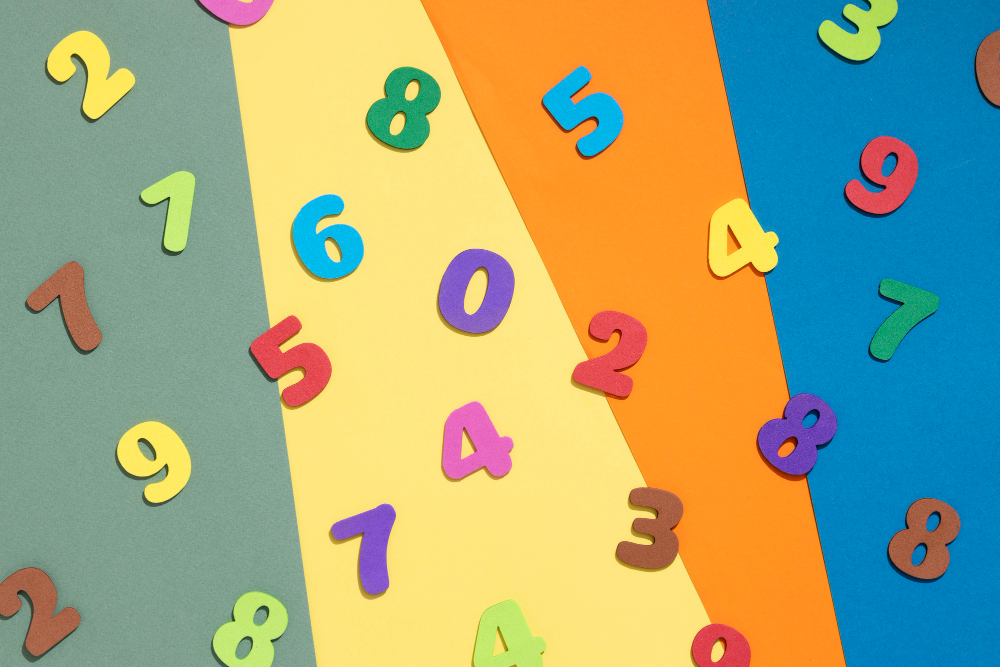# Teaching Place Value: Building the Foundation for Numerical Literacy - post

## Earn your Child Development Associate (CDA)

#### Teaching Place Value: Building the Foundation for Numerical LiteracyTeaching place value is a foundational concept in elementary #mathematics education, and it plays a significant role in shaping a child's mathematical understanding. Understanding place value is the key to deciphering how numbers are read and written, enabling young learners to make sense of the numerical world around them. In this article, we will delve into the importance of teaching place value, explore the base-ten system, and provide an engaging activity to reinforce these fundamental concepts in a childcare setting.

The Significance of Teaching Place Value

Teaching place value is a big deal in elementary math education. This fundamental concept provides the building blocks for young learners to comprehend numbers, perform operations, and solve mathematical problems effectively. Here's why it's so crucial:

• Numerical Literacy: Place value is essential for developing numerical literacy. It helps children grasp the structure of numbers, enabling them to read, write, and interpret numerical information accurately.
• Base-Ten System: Understanding place value is the gateway to the base-ten system, which is the foundation of our numerical system. It is critical for children to grasp this concept to manipulate numbers confidently.
• Problem Solving: Proficiency in place value is crucial for problem-solving and mathematical reasoning. It allows children to break down complex problems into manageable components and comprehend the logic behind mathematical operations.
• Math Confidence: Mastery of place value instills confidence in children as they navigate mathematical tasks. It empowers them to work with numbers effectively, which is crucial in their academic journey.

Understanding the Base-Ten System

At the heart of teaching place value is the concept of the base-ten system. In this numerical system, each digit's value depends on its position within the number. The key positions are the ones, tens, hundreds, thousands, and so on. Understanding these positional values is essential for young children to communicate numbers effectively.

To teach children the base-ten system, consider the following points:

• Ones: The rightmost digit represents ones. For example, in the number 27, the 7 signifies seven ones.
• Tens: The digit to the left of the ones represents tens. In the number 27, the 2 signifies two tens.
• Hundreds: The digit to the left of the tens represents hundreds. In the number 327, the 3 signifies three hundreds.
• Thousands and Beyond: Continue this pattern for larger numbers, with each digit's value based on its position.

Engaging Place Value Activity

To reinforce the concept of place value with school-age students in your childcare setting, consider using an interactive and hands-on activity. This activity involves a base-ten value chart and paper strips to reveal the number's value. Follow these instructions to engage your students effectively:

Materials Needed:

• Base-ten value chart (you can print or create one)
• Two paper strips
• A dry-erase marker (if you use a printed chart in a sheet protector)

Activity Steps:

• Introduce the Base-Ten System: Begin by explaining the base-ten system to your students. Discuss the significance of each position, from ones to tens and beyond. Use simple examples to illustrate how numbers are built based on this system.
• Prepare the Base-Ten Value Chart: Make sure you have a base-ten value chart ready for the activity. You can print one or create your own. Alternatively, place a printed chart in a sheet protector to allow for quick writing and erasing.
• Select a Number: Choose a number you want the students to work with, depending on their proficiency level. Start with smaller numbers and gradually progress to more complex ones.
• Use the Paper Strips: Take the two paper strips and place them on the chart. Begin with the rightmost strip, which represents the ones position, and move to the left. Position the strips accordingly to reveal the number's value.
• Involve the Students: Engage the students by encouraging them to guess and calculate the number's value. Start by asking questions like, "How many ones do you see?" "How many tens are there?" and "What's the complete number?"
• Discuss the Results: After the students have made their calculations and guesses, discuss the results with them. Ensure that they understand how each position contributes to the overall value of the number.
• Repeat with Different Numbers: Continue the activity with various numbers to reinforce the concept. You can gradually increase the complexity of the numbers as the students become more proficient.
• Encourage Participation: Promote active participation by having different students take turns in positioning the strips and explaining their calculations to the group.

Teaching place value is a fundamental component of elementary math education. It lays the groundwork for numerical literacy, problem-solving skills, and a strong mathematical foundation. By understanding the base-ten system and engaging in hands-on activities like the one described above, #educators can make learning place value an enjoyable and effective experience for young learners in childcare settings. So, take the opportunity to instill a solid grasp of place value in your students, and you'll be setting them on the path to mathematical success.

For more ways to support school-age children’s #development, register for one of ChildCareEd’s training courses below!